# 0708-1300/Homework Assignment 9

Announcements go here

## Contents

Read, reread and rereread your notes to this point, and make sure that you really, really really, really really really understand everything in them. Do the same every week!

## Doing

(Problems 1,2,4,5 below are taken with slight modifications from Hatcher's book, pages 79-80).

1. Show that if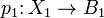$p_1\colon X_1\to B_1$ and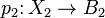$p_2\colon X_2 \to B_2$ are covering spaces, then so is their product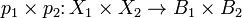$p_1\times p_2\colon X_1\times X_2\to B_1\times B_2$.
2. Construct (i.e., describe in explicit terms) a simply-connected covering space of the space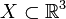$X\subset\mathbb{R}^3$ that is the union of a sphere and a diameter. Do the same when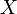$X$ is the union of a sphere and a circle intersecting it in two points.
3. Do the same to the space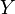$Y$ of the term test: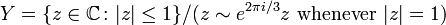$Y=\{z\in{\mathbb C}\colon|z|\leq 1\}/(z\sim e^{2\pi i/3}z\mbox{ whenever }|z|=1)$.
4. Find all the connected 2-sheeted and 3-sheeted covering spaces of the "figure eight space"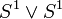$S^1\vee S^1$ (two circles joined at a point), up to isomorphism of covering spaces without base points.
5. Let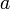$a$ and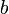$b$ be the generators of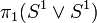$\pi_1(S^1\vee S^1)$ corresponding to the two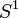$S^1$ summands. Draw a picture of the covering space of$S^1\vee S^1$ corresponding to the normal subgroup generated by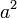$a^2$,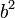$b^2$, and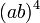$(ab)^4$, and prove that this covering space is indeed the correct one.

## Due Date

This assignment is due in class on Thursday February 28, 2008.

## Just for Fun

• What happens if in problem 1 we consider infinitely many covering spaces. That is, is the product of an infinite family of covering spaces a covering space?
• This raises another question. A "pathwise totally disconnected space" is a space in which every path is a constant path. How much of the theory of covering spaces can be generalized to "coverings" in which the fibers are pathwise totally disconnected, instead of discrete?